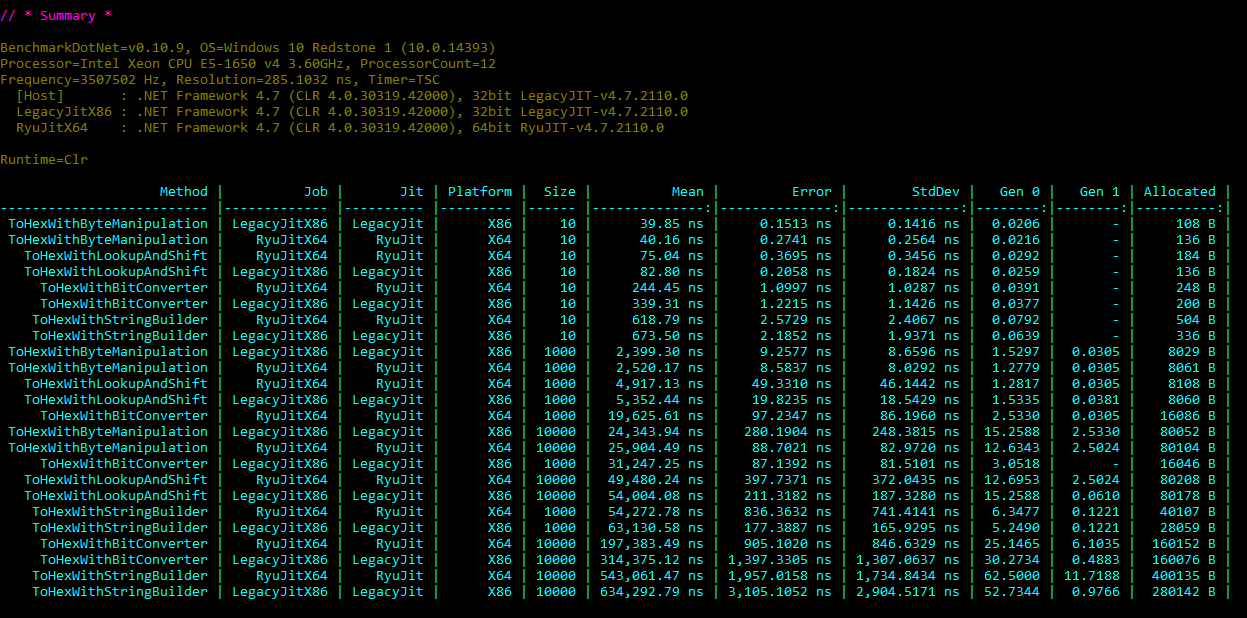# Comparing implementations with BenchmarkDotnet

• Gérald Barré

Sometimes you want to improve the performance of a function. So, you need to compare the performance of one or many different implementations to find the most performant in terms of time or/and memory. You can create a console application and use a Stopwatch to measure the time of each variant of your function. But then, how can you easily compare how your function behaves in x64 and x86, or on different runtimes. Are the execution of code well isolated? And why an implementation is better than the other?

To help you in this task, you can use BenchmarkDotNet, a powerful .NET library for benchmarking.Let's test BenchmarkDotNet with a simple function that converts a byte array to a hexadecimal string. We'll use 4 implementations coming from StackOverflow:

1. The basic implementation, often found on StackOverflow

``````public string ToHexWithStringBuilder(byte[] bytes)
{
var hex = new StringBuilder(bytes.Length * 2);
foreach (byte b in bytes)
hex.Append(b.ToString("X2"));
return hex.ToString();
}``````
2. Another implementation using `BitConverter`, a little bit shorter

``````public string ToHexWithBitConverter(byte[] bytes)
{
var hex = BitConverter.ToString(bytes);
return hex.Replace("-", "");
}``````
3. Another implementation with bit operations

``````public string ToHexWithLookupAndShift(byte[] bytes)
{
const string hexAlphabet = "0123456789ABCDEF";
var result = new StringBuilder(bytes.Length * 2);
foreach (byte b in bytes)
{
result.Append(hexAlphabet[b >> 4]);
result.Append(hexAlphabet[b & 0xF]);
}
return result.ToString();
}``````
4. The last one is trickier, but it works 😃

``````public string ToHexWithByteManipulation(byte[] bytes)
{
var c = new char[bytes.Length * 2];
int b;
for (int i = 0; i < bytes.Length; i++)
{
b = bytes[i] >> 4;
c[i * 2] = (char)(55 + b + (((b - 10) >> 31) & -7));
b = bytes[i] & 0xF;
c[i * 2 + 1] = (char)(55 + b + (((b - 10) >> 31) & -7));
}
return new string(c);
}``````

## #Using BenchmarkDotNet to compare the 4 implementations

First, create a console application. Add the following NuGet packages:

• `BenchmarkDotNet`
• `BenchmarkDotNet.Diagnostics.Windows`: provides additional data about runs

Then, create a class that contains the code to test, one method per implementation. Each method must be decorated by the `[Benchmark]` attribute. We want to test the implementation with different array sizes. BenchmarkDotNet provides a way to set parameters in the `[Params]` attribute. Let's see how it looks:

``````[OrderProvider(SummaryOrderPolicy.FastestToSlowest)] // Order the result
[RyuJitX64Job, LegacyJitX86Job] // Run with x64 and x86 runtimes
[MemoryDiagnoser] // Analyse the memory usage
public class ByteArrayToHexaBenchmark
{
// Initialize the byte array for each run
private byte[] _array;

[Params(10, 1000, 10000)]
public int Size { get; set; }

[GlobalSetup]
public void Setup()
{
_array = Enumerable.Range(0, Size).Select(i => (byte)i).ToArray();
}

// Code to benchmark
[Benchmark]
public string ToHexWithStringBuilder() => ToHexWithStringBuilder(_array);

[Benchmark]
public string ToHexWithBitConverter() => ToHexWithBitConverter(_array);

[Benchmark]
public string ToHexWithLookupAndShift() => ToHexWithLookupAndShift(_array);

[Benchmark]
public string ToHexWithByteManipulation() => ToHexWithByteManipulation(_array);

// Actual implementations
// code omitted for brevity... copy from above
}``````

Then, you run the benchmark:

``````public class Program
{
public static void Main()
{
BenchmarkRunner.Run<ByteArrayToHexaBenchmark>();
}
}``````

Now, you can run the application in release configuration to get the result:It's very easy to find the best implementation 😃

If you want to understand why a method behaves differently, you can use diagnosers. In the previous example, we use the `[MemoryDiagnoser]` attribute to get the memory used by each run. You can also use the `[InliningDiagnoser]` to determine if methods are inlined by the JIT. You can also get more advanced data using `[HardwareCounters]`. For instance, you can get the number of branch mispredictions. This gives you great insights on the behavior of your functions.

## #Conclusion

BenchmarkDotNet is very easy to set up, and gives you very accurate results in a few seconds. Thanks to the diagnosers, you can clearly understand how a function behaves at runtime, and take some actions to improve it. BenchmarkDotNet must be part of your toolbox.

Do you have a question or a suggestion about this post? Contact me!

Follow me: